# NCERT Solutions for Class 6 Maths Chapter 5 Understanding Elementary Shapes (Ex 5.3) Exercise 5.3

## NCERT Solutions for Class 6 Maths Chapter 5 Understanding Elementary Shapes (Ex 5.3) Exercise 5.3

Free PDF download of NCERT Solutions for Class 6 Maths Chapter 5 Exercise 5.3 (Ex 5.3) and all chapter exercises at one place prepared by expert teacher as per NCERT (CBSE) books guidelines. Class 6 Maths Chapter 5 Understanding Elementary Shapes Exercise 5.3 Questions with Solutions to help you to revise complete Syllabus and Score More marks. Register and get all exercise solutions in your emails.

Do you need help with your Homework? Are you preparing for Exams?
Study without Internet (Offline)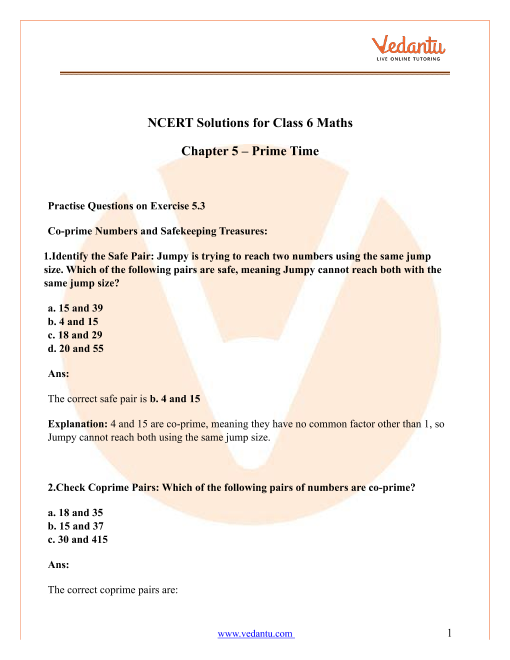Book your Free Demo session
Get a flavour of LIVE classes here at Vedantu## Access NCERT Solutions for Class 6 Maths Chapter - 5 - Understanding Elementary Shapes

Exercise - 5.3

1. Match the following:

 (i) Straight angle (a) Less than one-fourth a revolution (ii) Right angle (b) More than half a revolution (iii) Acute angle (c) Half of a revolution (iv) Obtuse angle (d) One-fourth a revolution (v) Reflex angle (e) Between $\dfrac{1}{4}$ and $\dfrac{1}{2}$ of a revolution (f) One complete revolution

Ans: We will match the given terms by looking at their definitions first and then matching them with the given options. The terms are matched as follows –

i. Straight Angle –

A straight angle is that angle which is formed over a straight line. We know that the measure of the angle over a straight line is $180^\circ$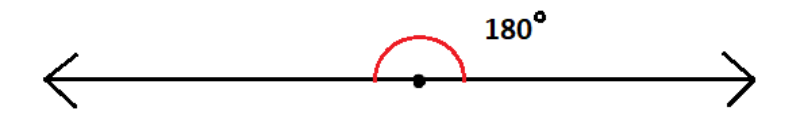Also, we know that the measure of an angle for a circle is $360^\circ$. This means that the measure for a complete revolution is $360^\circ$

So, as $180^\circ$ is half of $360^\circ$, it can be expressed as half of a revolution.

Therefore, option (i) matches with option (c).

ii. Right Angle –

A right angle is that angle which is formed between two perpendicular lines. We know that the measure of a right angle is $90^\circ$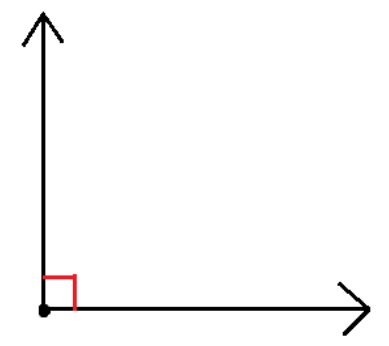Also, we know that the measure of an angle for a circle is $360^\circ$. This means that the measure for a complete revolution is $360^\circ$

So, as $90^\circ$ is one-fourth of $360^\circ$, it can be expressed as one-fourth of a revolution.

Therefore, option (ii) matches with option (d).

iii. Acute Angle –

An acute angle is that angle which measures between $0^\circ$ and $90^\circ$. In other words, the measure of an acute angle is less than $90^\circ$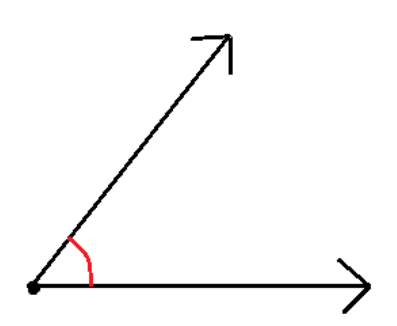Also, we know that the measure of an angle for a circle is $360^\circ$. This means that the measure for a complete revolution is $360^\circ$

So, as $90^\circ$ is one-fourth of $360^\circ$, it can be expressed as one-fourth of a revolution. Thus, less than $90^\circ$ can be expressed as less than one-fourth of a revolution.

Therefore, option (iii) matches with option (a).

iv. Obtuse Angle –

An obtuse angle is that angle which measures between $90^\circ$ and $180^\circ$. In other words, the measure of an obtuse angle is greater than $90^\circ$ and lesser than $180^\circ$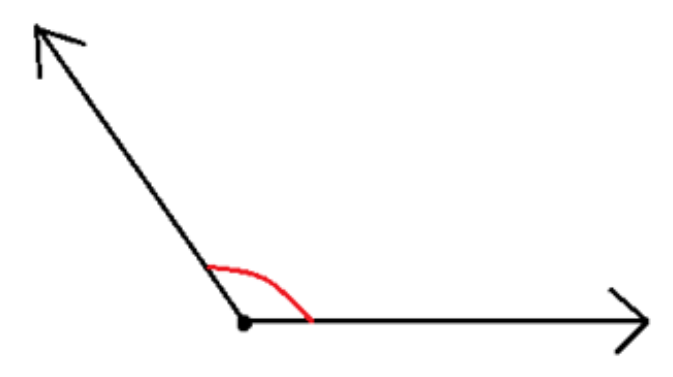Also, we know that the measure of an angle for a circle is $360^\circ$. This means that the measure for a complete revolution is $360^\circ$

So, as $90^\circ$ is one-fourth of $360^\circ$, it can be expressed as one-fourth of a revolution.

Also, as $180^\circ$ is half of $360^\circ$, it can be expressed as half of a revolution.

Thus, between $90^\circ$ and $180^\circ$ can be expressed as between $\dfrac{1}{4}$ and $\dfrac{1}{2}$ of a revolution.

Therefore, option (iv) matches with option (e).

v. Reflex Angle –

A reflex angle is that angle which has a measure of more than $180^\circ$.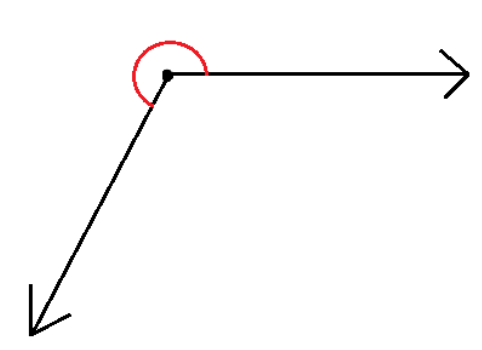Also, we know that the measure of an angle for a circle is $360^\circ$. This means that the measure for a complete revolution is $360^\circ$

So, as $180^\circ$ is half of $360^\circ$, the reflex angle can be expressed as more than half of a revolution.

Therefore, option (v) matches with option (b).

The matched pairs are as follows,

(i)-(c), (ii)-(d), (iii)-(a), (iv)-(e), and (v)-(b).

2. Classify each one of the following angles as right, straight, acute, obtuse or reflex.

(a)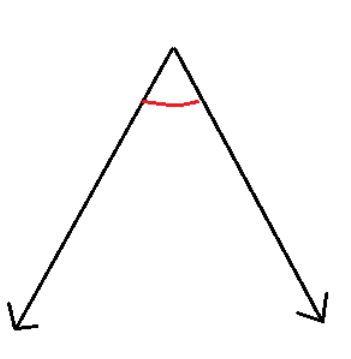Ans: From the given figure we can see that the measure of the given angle is less than $90^\circ$. Also, we know that the angles having measures less than $90^\circ$ are acute angles. Therefore, the angle given in the figure is classified as an acute angle.

(b)Ans: From the given figure we can see that the measure of the given angle is more than $90^\circ$. Also, we know that the angles having measures more than $90^\circ$ but less than $180^\circ$ are obtuse angles.

Therefore, the angle given in the figure is classified as an obtuse angle.

(c)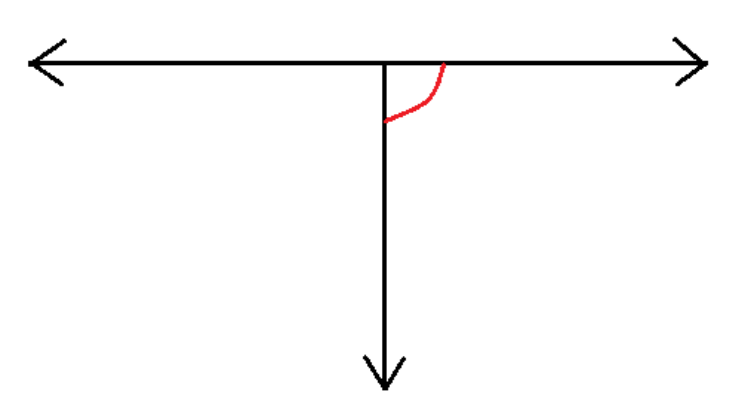Ans: From the given figure we can see that the measure of the given angle is $90^\circ$.

Also, we know that the angles having measures of $90^\circ$ are right angles.

Therefore, the angle given in the figure is classified as right angle.

(d)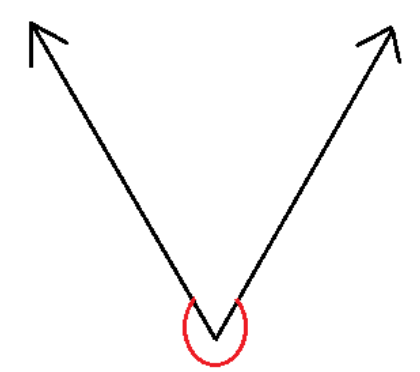Ans: From the given figure we can see that the measure of the given angle is greater than $180^\circ$.

Also, we know that the angles have measures greater than $180^\circ$ are reflex angles.

Therefore, the angle given in the figure is classified as reflex angle.

(e)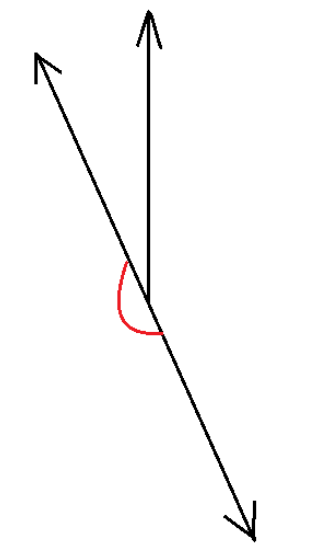Ans: From the given figure we can see that the measure of the given angle is $180^\circ$.

Also, we know that the angles having measures of $180^\circ$ are straight angles.

Therefore, the angle given in the figure is classified as straight angle.

(f)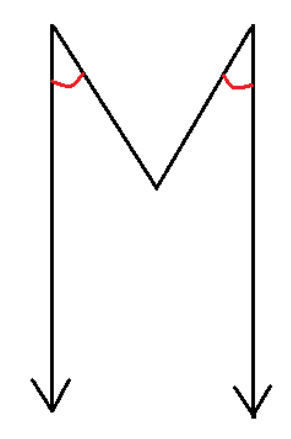Ans: From the given figure we can see that the measure of the given angles is less than $90^\circ$.

Also, we know that the angles having measures less than $90^\circ$ are acute angles.

Therefore, the angles given in the figure are classified as acute angles.

## NCERT Solutions for Class 6 Maths Chapter 5 Understanding Elementary Shapes Exercise 5.3

Opting for the NCERT solutions for Ex 5.3 Class 6 Maths is considered as the best option for the CBSE students when it comes to exam preparation. This chapter consists of many exercises. Out of which we have provided the Exercise 5.3 Class 6 Maths NCERT solutions on this page in PDF format. You can download this solution as per your convenience or you can study it directly from our website/ app online.

Vedantu in-house subject matter experts have solved the problems/ questions from the exercise with the utmost care and by following all the guidelines by CBSE. Class 6 students who are thorough with all the concepts from the Subject Understanding Elementary Shapes textbook and quite well-versed with all the problems from the exercises given in it, then any student can easily score the highest possible marks in the final exam. With the help of this Class 6 Maths Chapter 5 Exercise 5.3 solutions, students can easily understand the pattern of questions that can be asked in the exam from this chapter and also learn the marks weightage of the chapter. So that they can prepare themselves accordingly for the final exam.

Besides these NCERT solutions for Class 6 Maths Chapter 5 Exercise 5.3, there are plenty of exercises in this chapter which contain innumerable questions as well. All these questions are solved/answered by our in-house subject experts as mentioned earlier. Hence all of these are bound to be of superior quality and anyone can refer to these during the time of exam preparation. In order to score the best possible marks in the class, it is really important to understand all the concepts of the textbooks and solve the problems from the exercises given next to it.

Do not delay any more. Download the NCERT solutions for Class 6 Maths Chapter 5 Exercise 5.3 from Vedantu website now for better exam preparation. If you have the Vedantu app in your phone, you can download the same through the app as well. The best part of these solutions is these can be accessed both online and offline as well.SHARETWEETSHARESUBSCRIBE